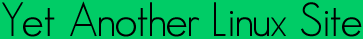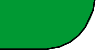Quick ?s
Cheat Sheets
Man Pages
The Lynx
Software
 ```RRDGRAPH_RPN(1) rrdtool RRDGRAPH_RPN(1) NAME rrdgraph_rpn - About RPN Math in rrdtool graph SYNOPSIS RPN expression:=vnameoperatorvalue[,RPN expression] DESCRIPTION If you have ever used a traditional HP calculator you already know RPN. The idea behind RPN is that you have a stack and push your data onto this stack. Whenever you execute an operation, it takes as many ele ments from the stack as needed. Pushing is done implicitly, so whenever you specify a number or a variable, it gets pushed onto the stack auto matically. At the end of the calculation there should be one and only one value left on the stack. This is the outcome of the function and this is what is put into the vname. For CDEF instructions, the stack is pro cessed for each data point on the graph. VDEF instructions work on an entire data set in one run. Note, that currently VDEF instructions only support a limited list of functions. Example: "VDEF:maximum=mydata,MAXIMUM" This will set variable "maximum" which you now can use in the rest of your RRD script. Example: "CDEF:mydatabits=mydata,8,*" This means: push variable mydata, push the number 8, execute the oper ator *. The operator needs two elements and uses those to return one value. This value is then stored in mydatabits. As you may have guessed, this instruction means nothing more than mydatabits = mydata * 8. The real power of RPN lies in the fact that it is always clear in which order to process the input. For expressions like "a = b + 3 * 5" you need to multiply 3 with 5 first before you add b to get a. However, with parentheses you could change this order: "a = (b + 3) * 5". In RPN, you would do "a = b, 3, +, 5, *" without the need for parentheses. OPERATORS Boolean operators LT, LE, GT, GE, EQ, NE Pop two elements from the stack, compare them for the selected con dition and return 1 for true or 0 for false. Comparing an unknown or an infinite value will always result in 0 (false). UN, ISINF Pop one element from the stack, compare this to unknown respec tively to positive or negative infinity. Returns 1 for true or 0 for false. IF Pops three elements from the stack. If the element popped last is 0 (false), the value popped first is pushed back onto the stack, otherwise the value popped second is pushed back. This does, indeed, mean that any value other than 0 is considered to be true. Example: "A,B,C,IF" should be read as "if (A) then (B) else (C)" Comparing values MIN, MAX Pops two elements from the stack and returns the smaller or larger, respectively. Note that infinite is larger than anything else. If one of the input numbers is unknown then the result of the opera tion will be unknown too. LIMIT Pops two elements from the stack and uses them to define a range. Then it pops another element and if it falls inside the range, it is pushed back. If not, an unknown is pushed. The range defined includes the two boundaries (so: a number equal to one of the boundaries will be pushed back). If any of the three numbers involved is either unknown or infinite this function will always return an unknown Example: "CDEF:a=alpha,0,100,LIMIT" will return unknown if alpha is lower than 0 or if it is higher than 100. Arithmetics +, -, *, /, % Add, subtract, multiply, divide, modulo SIN, COS, LOG, EXP, SQRT Sine and cosine (input in radians), log and exp (natural loga rithm), square root. ATAN Arctangent (output in radians). ATAN2 Arctangent of y,x components (output in radians). This pops one element from the stack, the x (cosine) component, and then a sec ond, which is the y (sine) component. It then pushes the arctan gent of their ratio, resolving the ambiguity between quadrants. Example: "CDEF:angle=Y,X,ATAN2,RAD2DEG" will convert "X,Y" compo nents into an angle in degrees. FLOOR, CEIL Round down or up to the nearest integer. DEG2RAD, RAD2DEG Convert angle in degrees to radians, or radians to degrees. Set Operations SORT, REV Pop one element from the stack. This is the count of items to be sorted (or reversed). The top count of the remaining elements are then sorted (or reversed) in place on the stack. Example: "CDEF:x=v1,v2,v3,v4,v5,v6,6,SORT,POP,5,REV,POP,+,+,+,4,/" will compute the average of the values v1 to v6 after removing the smallest and largest. AVG Pop one element (count) from the stack. Now pop count elements and build the average, ignoring all UNKNOWN values in the process. Example: "CDEF:x=a,b,c,d,4,AVG" TREND Create a "sliding window" average of another data series. Usage: CDEF:smoothed=x,1800,TREND This will create a half-hour (1800 second) sliding window average of x. The average is essentially computed as shown here: +---!---!---!---!---!---!---!---!---> now delay t0 <---------------> delay t1 <---------------> delay t2 <---------------> Value at sample (t0) will be the average between (t0-delay) and (t0) Value at sample (t1) will be the average between (t1-delay) and (t1) Value at sample (t2) will be the average between (t2-delay) and (t2) Special values UNKN Pushes an unknown value on the stack INF, NEGINF Pushes a positive or negative infinite value on the stack. When such a value is graphed, it appears at the top or bottom of the graph, no matter what the actual value on the y-axis is. PREV Pushes an unknown value if this is the first value of a data set or otherwise the result of this CDEF at the previous time step. This allows you to do calculations across the data. This function can not be used in VDEF instructions. PREV(vname) Pushes an unknown value if this is the first value of a data set or otherwise the result of the vname variable at the previous time step. This allows you to do calculations across the data. This function cannot be used in VDEF instructions. COUNT Pushes the number 1 if this is the first value of the data set, the number 2 if it is the second, and so on. This special value allows you to make calculations based on the position of the value within the data set. This function cannot be used in VDEF instructions. Time Time inside RRDtool is measured in seconds since the epoch. The epoch is defined to be "Thu Jan 1 00:00:00 UTC 1970". NOW Pushes the current time on the stack. TIME Pushes the time the currently processed value was taken at onto the stack. LTIME Takes the time as defined by TIME, applies the time zone offset valid at that time including daylight saving time if your OS sup ports it, and pushes the result on the stack. There is an elabo rate example in the examples section below on how to use this. Processing the stack directly DUP, POP, EXC Duplicate the top element, remove the top element, exchange the two top elements. VARIABLES These operators work only on VDEF statements. Note that currently ONLY these work for VDEF. MAXIMUM, MINIMUM, AVERAGE Return the corresponding value, MAXIMUM and MINIMUM also return the first occurrence of that value in the time component. Example: "VDEF:avg=mydata,AVERAGE" LAST, FIRST Return the last/first value including its time. The time for FIRST is actually the start of the corresponding interval, whereas LAST returns the end of the corresponding interval. Example: "VDEF:first=mydata,FIRST" TOTAL Returns the rate from each defined time slot multiplied with the step size. This can, for instance, return total bytes transfered when you have logged bytes per second. The time component returns the number of seconds. Example: "VDEF:total=mydata,TOTAL" PERCENT This should follow a DEF or CDEF vname. The vname is popped, another number is popped which is a certain percentage (0..100). The data set is then sorted and the value returned is chosen such that percentage percent of the values is lower or equal than the result. Unknown values are considered lower than any finite number for this purpose so if this operator returns an unknown you have quite a lot of them in your data. Infinite numbers are lesser, or more, than the finite numbers and are always more than the Unknown numbers. (NaN < -INF < finite values < INF) Example: "VDEF:perc95=mydata,95,PERCENT" LSLSLOPE, LSLINT, LSLCORREL Return the parameters for a Least Squares Line (y = mx +b) which approximate the provided dataset. LSLSLOPE is the slope (m) of the line related to the COUNT position of the data. LSLINT is the y-intercept (b), which happens also to be the first data point on the graph. LSLCORREL is the Correlation Coefficient (also know as Pearsons Product Moment Correlation Coefficient). It will range from 0 to +/-1 and represents the quality of fit for the approxima tion. Example: "VDEF:slope=mydata,LSLSLOPE" SEE ALSO rrdgraph gives an overview of how rrdtool graph works. rrdgraph_data describes DEF,CDEF and VDEF in detail. rrdgraph_rpn describes the RPN language used in the ?DEF statements. rrdgraph_graph page describes all of the graph and print functions. Make sure to read rrdgraph_examples for tips&tricks. AUTHOR Program by Tobias Oetiker This manual page by Alex van den Bogaerdt 1.2.15 2006-07-14 RRDGRAPH_RPN(1) ```Yals.net is © 1999-2009 Crescendo Communications Sharing tech info on the web for more than a decade! This page was generated Thu Apr 30 17:05:22 2009